×#### Thank you for registering.

One of our academic counsellors will contact you within 1 working day.

Click to Chat

1800-1023-196

+91-120-4616500

CART 0

• 0

MY CART (5)

Use Coupon: CART20 and get 20% off on all online Study Material

ITEM
DETAILS
MRP
DISCOUNT
FINAL PRICE
Total Price: Rs.

There are no items in this cart.
Continue Shopping• Complete JEE Main/Advanced Course and Test Series
• OFFERED PRICE: Rs. 15,900
• View Details

```Chapter 6: Algebraic Expressions and Identities Exercise – 6.5

Question: 1

Multiply

(5x + 3) by (7x + 2)

Solution:

To multiply, we will use distributive law as follows:

(5x + 3)(7x + 2)

= 5x(7x + 2)+ 3(7x + 2)

= (5x × 7x + 5x × 2) + (3 × 7x + 3 × 2)

= (35 x 2 + 10x) + (21x + 6)

= 35x2 + 10x + 21x + 6

= 35x2 + 31x + 6

Thus, the answer is 35x2 + 31x + 6

Question: 2

Multiply

(2x + 8) by (x – 3)

Solution:

To multiply, we will use distributive law as follows:Question: 3

Multiply

(7x + y) by (x + 5y)

Solution:

To multiply, we will use distributive law as follows:Question: 4

Multiply

(a – 1) by (0.1a2 + 3)

Solution:

To multiply, we will use distributive law as follows: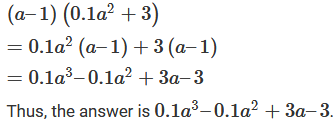Question: 5

Multiply

(3x2 + y2) (2x2 + 3y2)

Solution:

To multiply, we will use distributive law as follows:Question: 6

MultiplySolution:

To multiply, we will use distributive law as follows: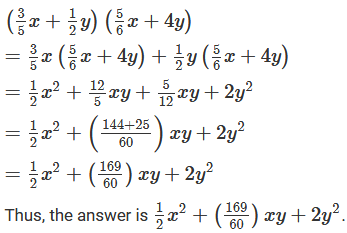Question: 7

Multiply

(x6 - y6)(x2 + y2)

Solution:

To multiply, we will use distributive law as follows: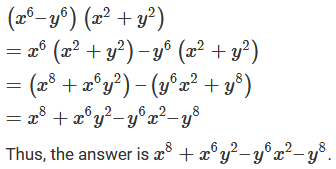Question: 8

Multiply

(x2 + y2) (3a + 2b)

Solution:

To multiply, we will use distributive law as follows:Question: 9

Multiply

[ – 3d + (–7f)](5d + f)

Solution:

To multiply, we will use distributive law as follows:Question: 10

Multiply

(0.8a – 0.5b)(1.5a – 3b)

Solution:

To multiply, we will use distributive law as follows: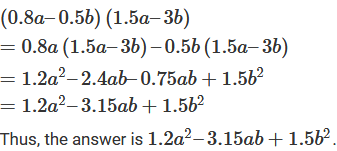Question: 11

Multiply

(2x2y2 – 5xy2)(x2 – y2)

Solution:

To multiply, we will use distributive law as follows:Question: 12

Multiply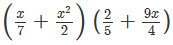Solution:

To multiply, we will use distributive law as follows: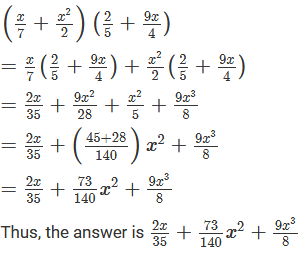Question: 13

MultiplySolution:

To multiply, we will use distributive law as follows: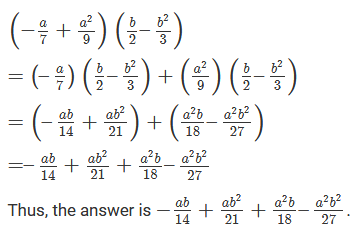Question: 14

Multiply

(3x2y – 5xy2)((1/5)x2 + (1/3)y2)

Solution:

To multiply, we will use distributive law as follows: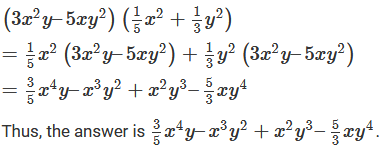Question: 16

Multiply

(2xy + 3y2)(3y2 – 2)

Solution:

To multiply, we will use distributive law as follows: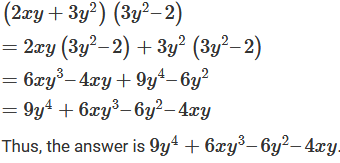Question: 17

Find the products and verify the result for x = -1 and y = -2:

(3x – 5y)(x + y)

Solution: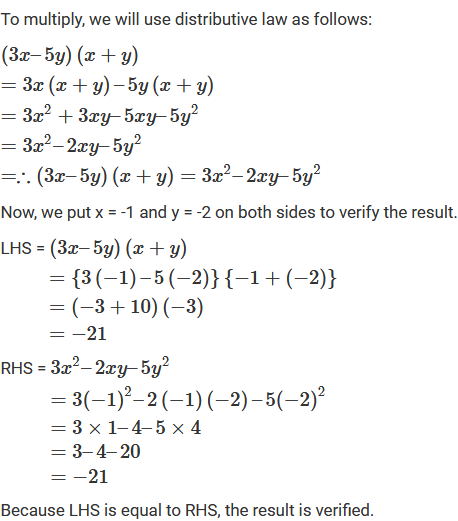Question: 18

Find the products and verify the result for x = -1 and y = – 2:

(x2y – 1)(3 – 2x2y)

Solution:

To multiply, we will use distributive law as follows: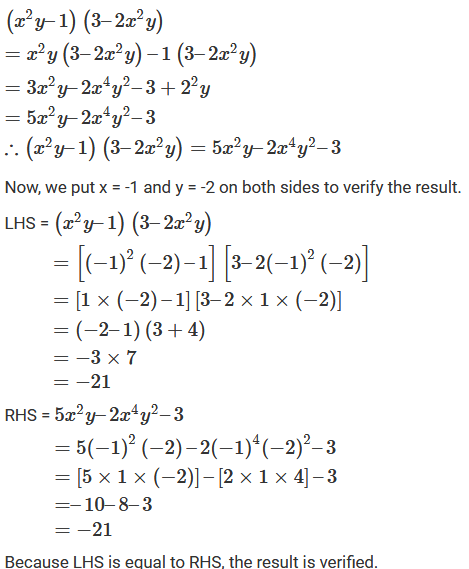Question: 19

Find the products and verify the result for x = -1 and y = -2:Solution:

To multiply, we will use distributive law as follows: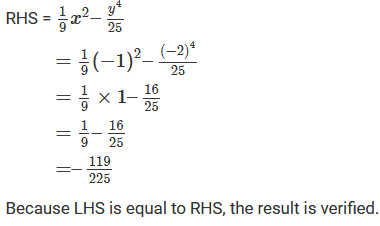Question: 20

Simplify

x2(x + 2y)(x – 3y)

Solution:

To simplify, we will use distributive law as follows: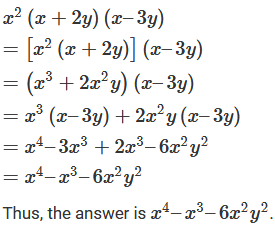Question: 21

Simplify

(x2 – 2y2)(x + 4y) x2y2

Solution:

To simplify, we will use distributive law as follows:Question: 22

Simplify

a2b2(a + 2b)(3a + b)

Solution:

To simplify, we will use distributive law as follows:Question: 23

Simplify

x2(x – y)y2(x + 2y)

Solution:

To simplify, we will use distributive law as follows: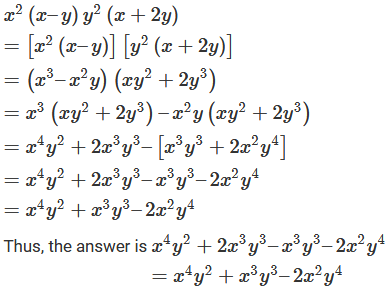Question: 24

Simplify

(x3 – 2x2 + 5x – 7)(2x – 3)

Solution:

To simplify, we will use distributive law as follows: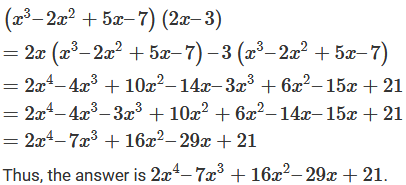Question: 25

Simplify

2x4 – 7x3 + 16x 2– 29x + 21

Solution:

To simplify, we will use distributive law as follows: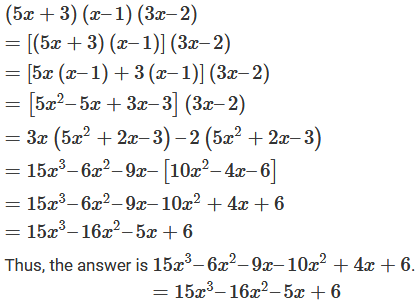Question: 26

Simplify

(5 – x)(6 – 5x)(2– x)

Solution:

To simplify, we will use distributive law as follows: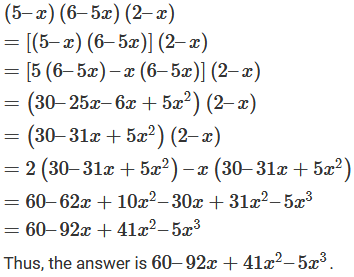Question: 27

Simplify

(2x2 + 3x – 5)(3x2 – 5x + 4)

Solution:

To simplify, we will use distributive law as follows:Question: 28

Simplify

(3x – 2)(2x – 3) + (5x – 3)(x + 1)

Solution:

To simplify, we will use distributive law as follows:Question: 29

Simplify

(5x – 3)(x + 2) – (2x + 5)(4x – 3)

Solution:

To simplify, we will use distributive law as follows: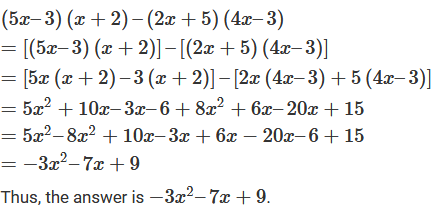Question: 30

Simplify

(3x + 2y)(4x + 3y) – (2x – y)(7x – 3y)

Solution:

To simplify, we will use distributive law as follows: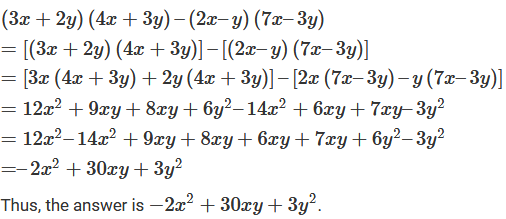Question: 31

Simplify

(x2 – 3x + 2)(5x – 2) – (3x2 + 4x – 5)(2x – 1)

Solution:

To simplify, we will use distributive law as follows: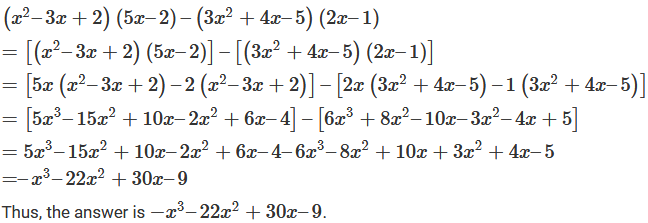Question: 32

Simplify

(x3 – 2x2 + 3x – 4)(x – 1) – (2x – 3)(x2 – x + 1)

Solution:

To simplify, we will use distributive law as follows: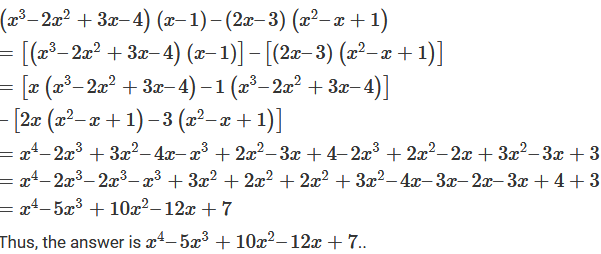```### Course Features

• 728 Video Lectures
• Revision Notes
• Previous Year Papers
• Mind Map
• Study Planner
• NCERT Solutions
• Discussion Forum
• Test paper with Video Solution